# Bayesian Zig Zag¶

Developing probabilistic models using grid methods and MCMC.

Thanks to Chris Fonnesback for his help with this notebook, and to Colin Carroll, who added features to pymc3 to support some of these examples.

In :
%matplotlib inline
%config InteractiveShell.ast_node_interactivity='last_expr_or_assign'

import numpy as np
import pymc3 as pm

import matplotlib.pyplot as plt


## Simulating hockey¶

I'll model hockey as a Poisson process, where each team has some long-term average scoring rate, lambda, in goals per game.

For the first example, I'll assume that lambda is somehow known to be 2.7. Since regulation play (as opposed to overtime) is 60 minutes, we can compute the goal scoring rate per minute.

In :
lam_per_game = 2.7
min_per_game = 60
lam_per_min = lam_per_game / min_per_game
lam_per_min, lam_per_min**2

Out:
(0.045000000000000005, 0.0020250000000000003)

If we assume that a goal is equally likely during any minute of the game, and we ignore the possibility of scoring more than one goal in the same minute, we can simulate a game by generating one random value each minute.

In :
def simulate_game(p, n=60):
goals = np.random.choice([0, 1], n, p=[1-p, p])
return np.sum(goals)


And simulate 10 games.

In :
size = 10
sample = [simulate_game(lam_per_min) for i in range(size)]

Out:
[1, 6, 2, 3, 0, 1, 3, 2, 2, 1]

If we simulate 1000 games, we can see what the distribution looks like. The average of this sample should be close to lam_per_game.

In :
size = 1000
sample_sim = [simulate_game(lam_per_min) for i in range(size)]
np.mean(sample_sim), lam_per_game

Out:
(2.706, 2.7)

## PMFs¶

To visualize distributions, I'll start with a probability mass function (PMF), which I'll implement using a Counter.

In :
from collections import Counter

class Pmf(Counter):

def normalize(self):
"""Normalizes the PMF so the probabilities add to 1."""
total = sum(self.values())
for key in self:
self[key] /= total

def sorted_items(self):
"""Returns the outcomes and their probabilities."""
return zip(*sorted(self.items()))


Here are some functions for plotting PMFs.

In :
plot_options = dict(linewidth=3, alpha=0.6)

def underride(options):
"""Add key-value pairs to d only if key is not in d.

options: dictionary
"""

for key, val in plot_options.items():
options.setdefault(key, val)
return options

def plot(xs, ys, **options):
"""Line plot with plot_options."""
plt.plot(xs, ys, **underride(options))

def bar(xs, ys, **options):
"""Bar plot with plot_options."""
plt.bar(xs, ys, **underride(options))

def plot_pmf(sample, **options):
"""Compute and plot a PMF."""
pmf = Pmf(sample)
pmf.normalize()
xs, ps = pmf.sorted_items()
bar(xs, ps, **options)

def decorate_pmf_goals():
"""Decorate the axes."""
plt.xlabel('Number of goals')
plt.ylabel('PMF')
plt.title('Distribution of goals scored')
legend()

def legend(**options):
"""Draw a legend only if there are labeled items.
"""
ax = plt.gca()
handles, labels = ax.get_legend_handles_labels()
if len(labels):
plt.legend(**options)


Here's what the results from the simulation look like.

In :
plot_pmf(sample_sim, label='simulation')
decorate_pmf_goals()

plt.savefig('zigzag1.png', dpi=150)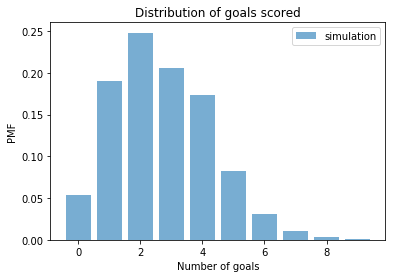## Poisson process¶

For large values of n, the process we just simulated converges to the Poisson distribution with parameter mu = n * p, which is also mu = lam_per_game.

We can use NumPy to generate a sample from a Poisson distribution.

In :
n = 60
p = lam_per_min
mu = n * p
sample_poisson = np.random.poisson(mu, size)
np.mean(sample_poisson)

Out:
2.733

And confirm that the results are similar to what we got from the model.

In :
plot_pmf(sample_sim, label='simulation')
plot_pmf(sample_poisson, label='Poisson')
decorate_pmf_goals()

plt.savefig('zigzag2.png', dpi=150)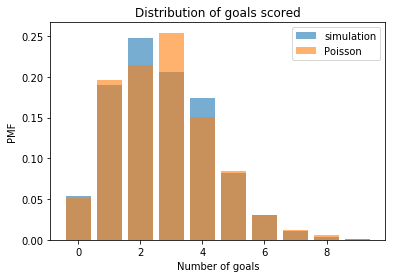But plotting PMFs is a bad way to compare distributions. It's better to use the cumulative distribution function (CDF).

In :
def plot_cdf(sample, **options):
"""Compute and plot the CDF of a sample."""
pmf = Pmf(sample)
xs, freqs = pmf.sorted_items()
ps = np.cumsum(freqs, dtype=np.float)
ps /= ps[-1]
plot(xs, ps, **options)

def decorate_cdf_rates():
"""Decorate the axes."""
plt.xlabel('Goal scoring rate (mu)')
plt.ylabel('CDF')
plt.title('Distribution of goal scoring rate')
legend()

def decorate_cdf_goals():
"""Decorate the axes."""
plt.xlabel('Number of goals')
plt.ylabel('CDF')
plt.title('Distribution of goals scored')
legend()

def plot_cdfs(*sample_seq, **options):
"""Plot multiple CDFs."""
for sample in sample_seq:
plot_cdf(sample, **options)
decorate_cdf_goals()


Comparing CDFs makes it clearer that the results from the simulation are consistent with the Poisson model.

In :
plot_cdf(sample_sim, label='simulation')
plot_cdf(sample_poisson, label='Poisson')
decorate_cdf_goals()

plt.savefig('zigzag3.png', dpi=150)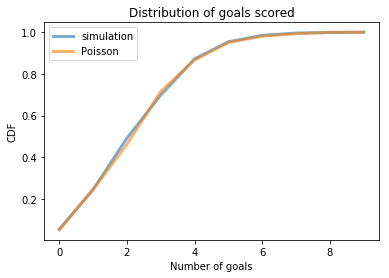## Warming up PyMC¶

Soon we will want to use pymc3 to do inference, which is really what it's for. But just to get warmed up, I will use it to generate a sample from a Poisson distribution.

In :
model = pm.Model()

with model:
goals = pm.Poisson('goals', mu)
trace = pm.sample_prior_predictive(1000)

In :
len(trace['goals'])

Out:
1000
In :
sample_pm = trace['goals']
np.mean(sample_pm)

Out:
2.634

This example is like using a cannon to kill a fly. But it help us learn to use the cannon.

In :
plot_cdf(sample_sim, label='simulation')
plot_cdf(sample_pm, label='PyMC')
decorate_cdf_goals()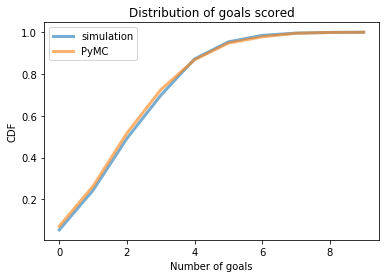## Evaluating the Poisson distribution¶

One of the nice things about the Poisson distribution is that we can compute its PMF analytically, and SciPy provides an implementation.

In :
from scipy.stats import poisson

xs = np.arange(11)
ps = poisson.pmf(xs, mu)
bar(xs, ps, label='Poisson PMF')
decorate_pmf_goals()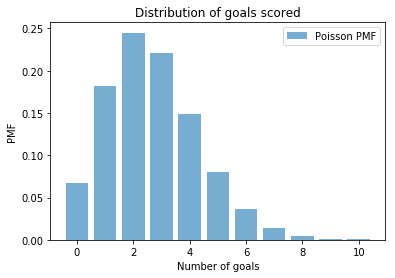And here's a function that computes the probability of scoring a given number of goals in a game, for a known value of mu.

In :
def poisson_likelihood(goals, mu):
"""Probability of goals given scoring rate.

goals: observed number of goals (scalar or sequence)
mu: hypothetical goals per game

returns: probability
"""
return np.prod(poisson.pmf(goals, mu))


Here's the probability of scoring 6 goals in a game if the long-term rate is 2.7 goals per game.

In :
poisson_likelihood(goals=6, mu=2.7)

Out:
0.036162211957124435

Here's the probability of scoring 3 goals.

In :
poisson_likelihood(goals=3, mu=2.7)

Out:
0.22046768454274915

This function also works with a sequence of goals, so we can compute the probability of scoring 6 goals in the first game and 3 in the second.

In :
poisson_likelihood(goals=[6, 3], mu=2.7)

Out:
0.007972599138131342

## Bayesian inference with grid approximation¶

Ok, it's finally time to do some inference! The function we just wrote computes the likelihood of the data, given a hypothetical value of mu:

$\mathrm{Prob}~(x ~|~ \mu)$

But what we really want is the distribution of mu, given the data:

$\mathrm{Prob}~(\mu ~|~ x)$

If only there were some theorem that relates these probabilities!

The following class implements Bayes's theorem.

In :
class Suite(Pmf):
"""Represents a set of hypotheses and their probabilities."""

def bayes_update(self, data, like_func):
"""Perform a Bayesian update.

data:      some representation of observed data
like_func: likelihood function that takes (data, hypo), where
hypo is the hypothetical value of some parameter,
and returns P(data | hypo)
"""
for hypo in self:
self[hypo] *= like_func(data, hypo)
self.normalize()

def plot(self, **options):
"""Plot the hypotheses and their probabilities."""
xs, ps = self.sorted_items()
plot(xs, ps, **options)

def decorate_pdf_rate():
"""Decorate the axes."""
plt.xlabel('Goals per game (mu)')
plt.ylabel('PDF')
plt.title('Distribution of goal scoring rate')
legend()


I'll start with a uniform prior just to keep things simple. We'll choose a better prior later.

In :
hypo_mu = np.linspace(0, 15, num=51)
hypo_mu

Out:
array([ 0. ,  0.3,  0.6,  0.9,  1.2,  1.5,  1.8,  2.1,  2.4,  2.7,  3. ,
3.3,  3.6,  3.9,  4.2,  4.5,  4.8,  5.1,  5.4,  5.7,  6. ,  6.3,
6.6,  6.9,  7.2,  7.5,  7.8,  8.1,  8.4,  8.7,  9. ,  9.3,  9.6,
9.9, 10.2, 10.5, 10.8, 11.1, 11.4, 11.7, 12. , 12.3, 12.6, 12.9,
13.2, 13.5, 13.8, 14.1, 14.4, 14.7, 15. ])

Initially uniform_prior represents the prior distribution of mu.

In :
uniform_prior = Suite(hypo_mu)
uniform_prior.normalize()
uniform_prior.plot(label='prior')
decorate_pdf_rate()

plt.savefig('zigzag4.png', dpi=150)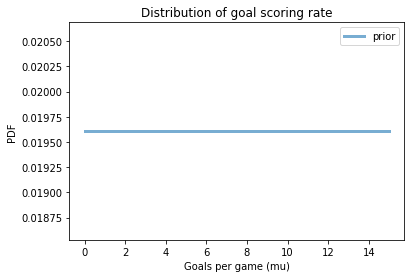Now we can update it with the data and plot the posterior.

In :
uniform_prior.bayes_update(data=3, like_func=poisson_likelihood)
uniform_prior.plot(label='posterior')
decorate_pdf_rate()

plt.savefig('zigzag5.png', dpi=150)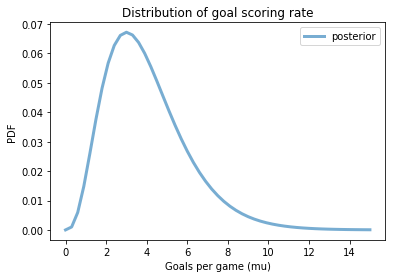With a uniform prior, the posterior is the likelihood function, and the MAP is the value of mu that maximizes likelihood, which is the observed number of goals, 6.

This result is probably not reasonable, because the prior was not reasonable.

## A better prior¶

To construct a better prior, I'll use a Gamma distribution with parameters chosen to be consistent with previous playoff games.

We can use make_gamma_dist to construct a prior suite with the given parameters.

In :
from scipy.stats import gamma

def make_gamma_suite(xs, alpha, beta):
"""Makes a suite based on a gamma distribution.

xs: places to evaluate the PDF
alpha, beta: parameters of the distribution

returns: Suite
"""
ps = gamma(a=alpha, scale=1/beta).pdf(xs)
prior = Suite(dict(zip(xs, ps)))
prior.normalize()
return prior


Here's what it looks like.

In :
alpha = 9
beta = 3
hypo_mu = np.linspace(0, 15, num=101)

gamma_prior = make_gamma_suite(hypo_mu, alpha, beta)

gamma_prior.plot(label='gamma prior')
decorate_pdf_rate()

plt.savefig('zigzag6.png', dpi=150)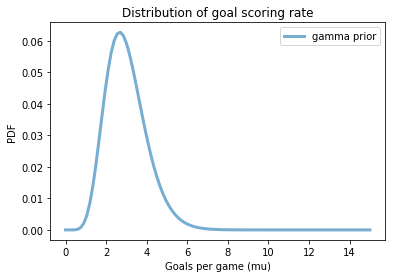And we can update this prior using the observed data. If we only score 1 goal in a game, our estimate for the goal scoring rate shifts left.

In :
posterior = gamma_prior.copy()
posterior.bayes_update(data=1, like_func=poisson_likelihood)

gamma_prior.plot(label='prior')
posterior.plot(label='posterior')
decorate_pdf_rate()

plt.savefig('zigzag7.png', dpi=150)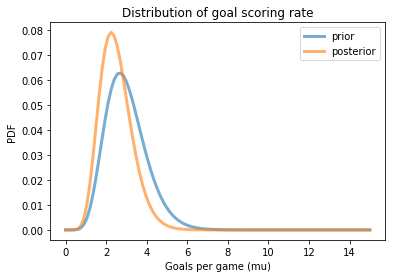If we go back to the original prior, and score 6 goals in a game, the estimate shifts to the right.

In :
posterior = gamma_prior.copy()
posterior.bayes_update(data=6, like_func=poisson_likelihood)

gamma_prior.plot(label='prior')
posterior.plot(label='posterior')
decorate_pdf_rate()

plt.savefig('zigzag8.png', dpi=150)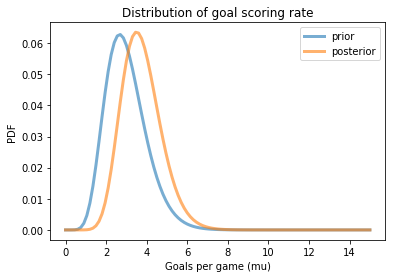If we score 3 goals in a game, the estimate doesn't shift much, but it gets pointier, which means we are more certain about the goal scoring rate.

In :
posterior = gamma_prior.copy()
posterior.bayes_update(data=3, like_func=poisson_likelihood)

gamma_prior.plot(label='prior')
posterior.plot(label='posterior')
decorate_pdf_rate()

plt.savefig('zigzag8.png', dpi=150)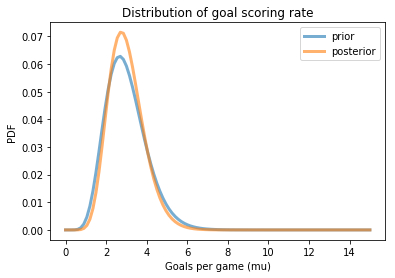## Posterior predictive distribution¶

Now, let's get to what is usually the point of this whole exercise, making predictions.

The prior represents what we believe about the distribution of mu based on the data (and our prior beliefs).

Each value of mu is a possible goal scoring rate.

For a given value of mu, we can generate a distribution of goals scored in a particular game, which is Poisson.

But we don't have a given value of mu, we have a whole bunch of values for mu, with different probabilities.

So the posterior predictive distribution is a mixture of Poissons with different parameters.

The simplest way to generate the posterior predictive distribution is to

1. Draw a random mu from the posterior distribution.

2. Draw a random number of goals from Poisson(mu).

3. Repeat.

Here's a function that draws a sample from a posterior Suite:

In :
def sample_suite(suite, size):
"""Draw a random sample from a Suite

suite: Suite object
size: sample size
"""
xs, ps = np.transpose(list(suite.items()))
return np.random.choice(xs, size, replace=True, p=ps)


Here's a sample of mu drawn from the posterior distribution (after one game).

In :
size = 10000
sample_post = sample_suite(posterior, size)
np.mean(sample_post)

Out:
2.988255

Now for each value of mu in the posterior sample we draw one sample from Poisson(mu)

In :
sample_post_pred = np.random.poisson(sample_post)
np.mean(sample_post_pred)

Out:
2.9984

The result is a sample from the posterior predictive distribution. Here's what it looks like.

In :
plot_pmf(sample_post_pred, label='posterior predictive sample')
decorate_pmf_goals()

plt.savefig('zigzag9.png', dpi=150)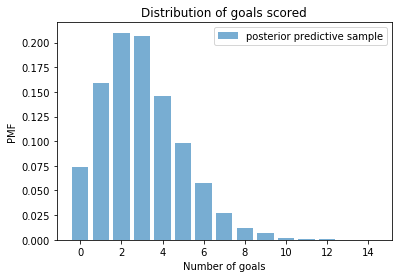The prior and posterior are distributions of mu, which is a continuous value.

The prior predictive and posterior predictive are distributions of goals, which are discrete.

To help keep them straight, I will plot distributions of mu as CDFs, and distributions of goals as PMFs.

## Back to PyMC¶

Previously we used PyMC to draw a sample from a Poisson distribution with known mu.

Now we'll use it to draw a sample from a gamma distribution of mu, with known alpha and beta.

And then use that value of mu to draw a sample from a Poisson distribution.

Here are the values of the parameters:

In :
print(alpha, beta)

9 3


Here's what the model looks like:

In :
model = pm.Model()

with model:
mu = pm.Gamma('mu', alpha, beta)
goals = pm.Poisson('goals', mu)
trace = pm.sample_prior_predictive(1000)


The distribution of mu from this model is the prior. Let's see what it looks like:

In :
sample_prior_pm = trace['mu']
np.mean(sample_prior_pm)

Out:
2.9737837778840777

And compare it to a sample from the gamma prior.

In :
sample_prior = sample_suite(gamma_prior, 1000)
np.mean(sample_prior)

Out:
2.9865
In :
plot_cdf(sample_prior, label='prior')
plot_cdf(sample_prior_pm, label='prior pymc')
decorate_cdf_rates()

plt.savefig('zigzag10.png', dpi=150)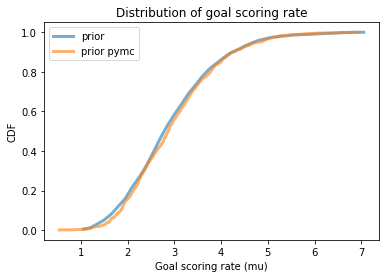It looks pretty good.

The distributions of goals from this model is the prior predictive.

In :
sample_prior_pred_pm = trace['goals']
np.mean(sample_prior_pred_pm)

Out:
2.965

And let's compare it to a prior predictive distribution estimated by sampling.

In :
sample_prior_pred = np.random.poisson(sample_prior)
np.mean(sample_prior_pred)

Out:
2.971
In :
plot_cdf(sample_prior_pred, label='prior pred')
plot_cdf(sample_prior_pred_pm, label='prior pred pymc')
decorate_cdf_goals()

plt.savefig('zigzag11.png', dpi=150)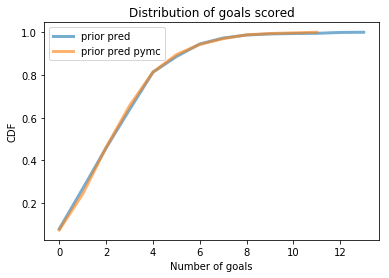Looks good.

## Now with PyMC¶

Finally, we are ready to use PyMC for actual inference. We just have to make one small change.

Instead of generating goals, we'll mark goals as observed and provide the observed data, 3.

And instead of called sample_prior_predictive, we'll call sample, which is understood to sample from the posterior distribution of mu.

In :
model = pm.Model()

with model:
mu = pm.Gamma('mu', alpha, beta)
goals = pm.Poisson('goals', mu, observed=3)
trace = pm.sample(1000, tune=2000)

Auto-assigning NUTS sampler...
Multiprocess sampling (4 chains in 4 jobs)
NUTS: [mu]
Sampling 4 chains: 100%|██████████| 12000/12000 [00:03<00:00, 3506.74draws/s]
The acceptance probability does not match the target. It is 0.6927188582727735, but should be close to 0.8. Try to increase the number of tuning steps.


With goals fixed, the only unknown is mu, so trace contains a sample drawn from the posterior distribution of mu. We can plot the posterior using a function provided by PyMC:

In :
pm.plot_posterior(trace)
decorate_pdf_rate()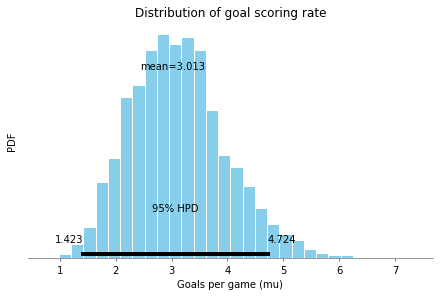And we can extract a sample from the posterior of mu

In :
sample_post_pm = trace['mu']
np.mean(sample_post_pm)

Out:
3.0131639332686024

And compare it to the sample we drew from the grid approximation:

In :
plot_cdf(sample_post, label='posterior grid')
plot_cdf(sample_post_pm, label='posterior pymc')
decorate_cdf_rates()

plt.savefig('zigzag12.png', dpi=150)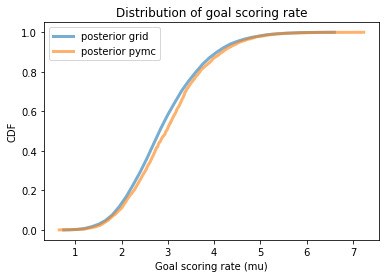Again, it looks pretty good.

To sample from the posterior predictive distribution, we can use sample_posterior_predictive:

In :
with model:
post_pred = pm.sample_posterior_predictive(trace, samples=1000)

100%|██████████| 1000/1000 [00:00<00:00, 2746.40it/s]


Here's what it looks like:

In :
sample_post_pred_pm = post_pred['goals']
sample_post_pred_pm.shape

Out:
(1000,)
In :
sample_post_pred_pm = post_pred['goals']
np.mean(sample_post_pred_pm)

Out:
3.092
In :
plot_cdf(sample_post_pred, label='posterior pred grid')
plot_cdf(sample_post_pred_pm, label='posterior pred pm')
decorate_cdf_goals()

plt.savefig('zigzag13.png', dpi=150)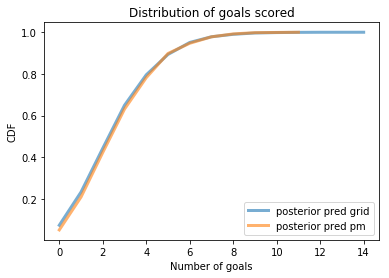Looks pretty good!

## Two teams¶

We can extend the model to estimate different values of mu for the two teams.

In :
model = pm.Model()

with model:
mu_BOS = pm.Gamma('mu_BOS', alpha, beta)
mu_ANA = pm.Gamma('mu_ANA', alpha, beta)
goals_BOS = pm.Poisson('goals_BOS', mu_BOS, observed=3)
goals_ANA = pm.Poisson('goals_ANA', mu_ANA, observed=1)
trace = pm.sample(1000, tune=2000)

Auto-assigning NUTS sampler...
Multiprocess sampling (4 chains in 4 jobs)
NUTS: [mu_ANA, mu_BOS]
Sampling 4 chains: 100%|██████████| 12000/12000 [00:03<00:00, 3790.51draws/s]


We can use traceplot to review the results and do some visual diagnostics.

In :
pm.traceplot(trace);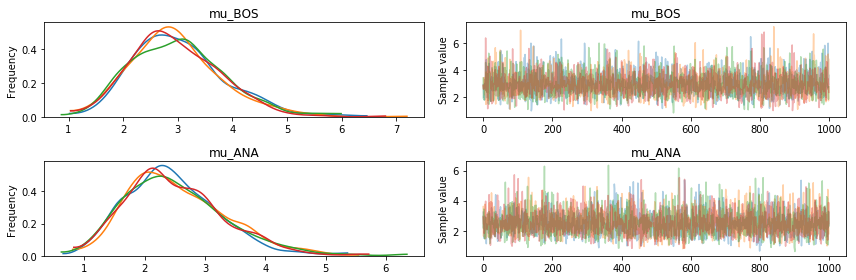Here are the posterior distribitions for mu_BOS and mu_ANA.

In :
mu_BOS = trace['mu_BOS']
plot_cdf(mu_BOS, label='mu_BOS posterior')

mu_ANA = trace['mu_ANA']
plot_cdf(mu_ANA, label='mu_ANA posterior')

decorate_cdf_rates()
np.mean(mu_BOS), np.mean(mu_ANA)

plt.savefig('zigzag14.png', dpi=150)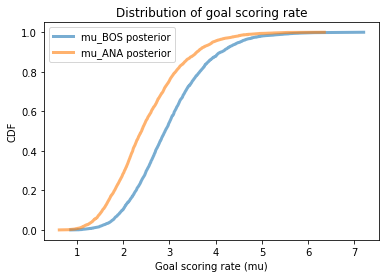On the basis of one game here's the probability that Boston is the better team.

In :
np.mean(mu_BOS > mu_ANA)

Out:
0.66725
In :
np.mean(mu_BOS == mu_ANA)

Out:
0.0

## Predictions¶

Even if Boston is the better team, that doesn't mean they'll win the next game.

We can use sample_posterior_predictive to generate predictions.

In :
with model:
post_pred = pm.sample_posterior_predictive(trace, samples=1000)

100%|██████████| 1000/1000 [00:00<00:00, 1386.94it/s]


Here are the posterior predictive distributions of goals scored.

In :
goals_BOS = post_pred['goals_BOS'].flatten()
goals_ANA = post_pred['goals_ANA'].flatten()

plot_cdf(goals_BOS, label='BOS')
plot_cdf(goals_ANA, label='ANA')
decorate_cdf_goals()

plt.savefig('zigzag15.png', dpi=150)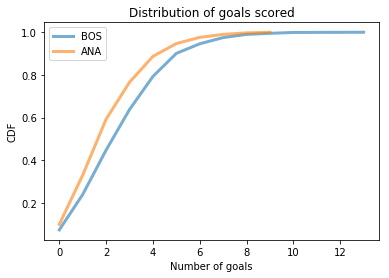Here's the chance that Boston wins the next game.

In :
win = np.mean(goals_BOS > goals_ANA)
win

Out:
0.513

The chance that they lose.

In :
lose = np.mean(goals_ANA > goals_BOS)
lose

Out:
0.335

And the chance of a tie.

In :
tie = np.mean(goals_BOS == goals_ANA)
tie

Out:
0.152

## Overtime!¶

In the event of a tie, the teams play a five-minute overtime period during which the team that scores first wins.

In a Poisson process with rate parameter mu, the time until the next event is exponential with parameter lam = 1/mu.

So we can take a sample from the posterior distributions of mu:

In :
mu_ANA = trace['mu_ANA']
mu_BOS = trace['mu_BOS']

Out:
array([2.94596135, 2.56428703, 2.02257676, ..., 3.16156756, 3.00181319,
2.37807074])

And generate time to score,tts, for each team:

In :
tts_ANA  = np.random.exponential(1/mu_ANA)
np.mean(tts_ANA)

Out:
0.4452535794803446
In :
tts_BOS  = np.random.exponential(1/mu_BOS)
np.mean(tts_BOS)

Out:
0.35965728049629286

Here's the chance that Boston wins in overtime.

In :
win_ot = np.mean(tts_BOS < tts_ANA)
win_ot

Out:
0.5475

Since tts is continuous, ties are unlikely.

In :
total_win = win + tie * win_ot
total_win

Out:
0.59622